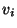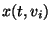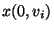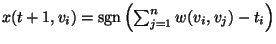Next: MAXIMUM CHANNEL ASSIGNMENT Up: Miscellaneous Previous: MAXIMUM STRING FOLDING   Index

### MINIMUM ATTRACTION RADIUS FOR BINARY HOPFIELD NET

• INSTANCE: An n-node synchronous binary Hopfield network and a stable initial vector of states. A binary Hopfield network is a complete graph where each edge has an integer weightand each vertex has an integer threshold value. At each time step t each vertexhas a state.is given by u andwhereis the sign function. An initial vector of states is stable ifeventually converges for all i.

• SOLUTION: An initial vector of states v that either converges to a different vector than u or is not stable.

• MEASURE: The Hamming distance between u and v. If v is the vector nearest to u that does not converge to the same vector as u, then this distance is the attraction radius.

• Bad News: Not approximable withinfor any.

• Comment: Transformation from MINIMUM INDEPENDENT DOMINATING SET.Next: MAXIMUM CHANNEL ASSIGNMENT Up: Miscellaneous Previous: MAXIMUM STRING FOLDING   Index
Viggo Kann
2000-03-20